# CLASS 12 PHYSICS CHAPTER 13- NUCLEI

Question1. (a) Two stable isotopes of lithium and have respective abundances of 7.5% and 92.5%. These isotopes have masses 6.01512 u and 7.01600 u, respectively. Find the atomic mass of lithium.

(b) Boron has two stable isotopes, and. Their respective masses are 10.01294 u and 11.00931 u, and the atomic mass of boron is 10.811 u. Find the abundances of and .

Solution :
(a) Mass of lithium isotope , m1 = 6.01512 u

Mass of lithium isotope , m2 = 7.01600 u

Abundance of , η1= 7.5%

Abundance of , η2= 92.5%

The atomic mass of lithium atom is given as:

=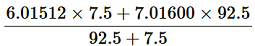= 6.940934 u.

(b) Mass of boron isotope , m1 = 10.01294 u

Mass of boron isotope , m2 = 11.00931 u

Abundance of , η1 = x%

Abundance of , η2= (100 − x)%

Atomic mass of boron, m = 10.811 u

The atomic mass of boron atom is given as:

x = 19.821/0.99637 = 19.89%

And 100 − x = 80.11%

Hence, the abundance of is 19.89% and that of is 80.11%.

Question2. The three stable isotopes of neon: , and have respective abundances of 90.51%, 0.27% and 9.22%. The atomic masses of the three isotopes are 19.99 u, 20.99 u and 21.99 u, respectively. Obtain the average atomic mass of neon.

Solution :
Atomic mass of , m1= 19.99 u

Abundance of , η= 90.51%

Atomic mass of , m= 20.99 u

Abundance of , η= 0.27%

Atomic mass of , m= 21.99 u

Abundance of , η3 = 9.22%

The average atomic mass of neon is given as:

= 20.1771u

Question3. Obtain the binding energy (in MeV) of a nitrogen nucleus, given =14.00307 u

Solution :
Atomic mass of nitrogen, m = 14.00307 u

A nucleus of nitrogen contains 7 protons and 7 neutrons.

Hence, the mass defect of this nucleus, Δm = 7mH + 7mn − m

Where,

Mass of a proton, mH = 1.007825 u

Mass of a neutron, mn= 1.008665 u

∴Δm = 7 × 1.007825 + 7 × 1.008665 − 14.00307

= 7.054775 + 7.06055 − 14.00307

= 0.11236 u

But 1 u = 931.5 MeV/c2

∴Δm = 0.11236 × 931.5 MeV/c2

Hence, the binding energy of the nucleus is given as:

Eb = Δmc2

Where,

c = Speed of light

∴E= 0.11236 × 931.5 (MeV/c2)

= 104.66334 MeV

Hence, the binding energy of a nitrogen nucleus is 104.66334 MeV.

Question4. Obtain the binding energy of the nuclei and in units of MeV from the following data:

m= 55.934939 u  m= 208.980388 u

Solution :
Atomic mass of, m1 = 55.934939 u

nucleus has 26 protons and (56 − 26) = 30 neutrons

Hence, the mass defect of the nucleus, Δm = 26 × mH + 30 × mn − m1

Where,

Mass of a proton, mH = 1.007825 u

Mass of a neutron, mn = 1.008665 u

∴Δm = 26 × 1.007825 + 30 × 1.008665 − 55.934939

= 26.20345 + 30.25995 − 55.934939

= 0.528461 u

But 1 u = 931.5 MeV/c2

∴Δm = 0.528461 × 931.5 MeV/c2

The binding energy of this nucleus is given as:

Eb1 = Δmc2

Where,

c = Speed of light

∴Eb1 = 0.528461 × 931.5 (MeV/c2)

= 492.26 MeV

Average binding energy per nucleon 492.26/56 = 8.79 MeV

Atomic mass of, m2 = 208.980388 u

nucleus has 83 protons and (209 − 83) 126 neutrons.

Hence, the mass defect of this nucleus is given as:

Δm’ = 83 × mH + 126 × mn − m2

Where,

Mass of a proton, mH = 1.007825 u

Mass of a neutron, mn = 1.008665 u

∴Δm’ = 83 × 1.007825 + 126 × 1.008665 − 208.980388

= 83.649475 + 127.091790 − 208.980388

= 1.760877 u

But 1 u = 931.5 MeV/c2

∴Δm’ = 1.760877 × 931.5 MeV/c2

Hence, the binding energy of this nucleus is given as:

Eb2 = Δm’c2

= 1.760877 × 931.5(MeV/c2)

= 1640.26 MeV

Average bindingenergy per nucleon = 1640.26/209 = 7.848 MeV

Question5. A given coin has a mass of 3.0 g. Calculate the nuclear energy that would be required to separate all the neutrons and protons from each other. For simplicity assume that the coin is entirely made of atoms (of mass 62.92960 u).

Solution :
Mass of a copper coin, m’ = 3 g

Atomic mass of atom, m = 62.92960 u

The total number of atoms in the coin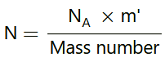Where,

NA = Avogadro’s number = 6.023 × 1023 atoms /g

Mass number = 63 g

nucleus has 29 protons and (63 − 29) 34 neutrons

∴Mass defect of this nucleus, Δm’ = 29 × mH + 34 × mn − m

Where,

Mass of a proton, mH = 1.007825 u

Mass of a neutron, mn = 1.008665 u

∴Δm’ = 29 × 1.007825 + 34 × 1.008665 − 62.9296

= 0.591935 u

Mass defect of all the atoms present in the coin, Δm = 0.591935 × 2.868 × 1022

= 1.69766958 × 1022 u

But 1 u = 931.5 MeV/c2

∴Δm = 1.69766958 × 1022 × 931.5 MeV/c2

Hence, the binding energy of the nuclei of the coin is given as:

Eb= Δmc2

= 1.69766958 × 1022 × 931.5 (MeV/c2)

= 1.581 × 1025 MeV

But 1 MeV = 1.6 × 10−13 J

Eb = 1.581 × 1025 × 1.6 × 10−13

= 2.5296 × 1012 J

This much energy is required to separate all the neutrons and protons from the given coin.

Question6. Write nuclear reaction equations for

(i) α-decay of (ii) α-decay of

(iii) β-decay of (iv) β-decay of

(v) β+-decay of (vi) β+-decay of

(vii) Electron capture of

Solution :
α is a nucleus of helium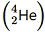and β is an electron (e− for β and e+ for β+). In every α-decay, there is a loss of 2 protons and 4 neutrons. In every β+-decay, there is a loss of 1 proton and a neutrino is emitted from the nucleus. In every β-decay, there is a gain of 1 proton and an antineutrino is emitted from the nucleus.

For the given cases, the various nuclear reactions can be written as:

Question7. A radioactive isotope has a half-life of T years. How long will it take the activity to reduce to a) 3.125%, b) 1% of its original value?

Solution :
Half-life of the radioactive isotope = T years

Original amount of the radioactive isotope = N0

(a) After decay, the amount of the radioactive isotope = N

It is given that only 3.125% of Nremains after decay. Hence, we can write:

Where,

λ = Decay constant

t = Time

Hence, the isotope will take about 5T years to reduce to 3.125% of its original value.

(b) After decay, the amount of the radioactive isotope = N

It is given that only 1% of Nremains after decay. Hence, we can write:

Hence, the isotope will take about 6.645T years to reduce to 1% of its original value.

Question8. The normal activity of living carbon-containing matter is found to be about 15 decays per minute for every gram of carbon. This activity arises from the small proportion of radioactive present with the stable carbon isotope . When the organism is dead, its interaction with the atmosphere (which maintains the above equilibrium activity) ceases and its activity begins to drop. From the known half-life (5730 years) of, and the measured activity, the age of the specimen can be approximately estimated. This is the principle of dating used in archaeology. Suppose a specimen from Mohenjodaro gives an activity of 9 decays per minute per gram of carbon. Estimate the approximate age of the Indus-Valley civilisation.

Solution :
Decay rate of living carbon-containing matter, R = 15 decay/min

Let N be the number of radioactive atoms present in a normal carbon- containing matter.

Half life of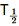= 5730 years

The decay rate of the specimen obtained from the Mohenjodaro site:

R’ = 9 decays/min

Let N’ be the number of radioactive atoms present in the specimen during the Mohenjodaro period.

Therefore, we can relate the decay constant, λand time, t as:

Hence, the approximate age of the Indus-Valley civilisation is 4223.5 years.

Question9. Obtain the amount of necessary to provide a radioactive source of 8.0 mCi strength. The half-life of is 5.3 years.

Solution :
The strength of the radioactive source is given as:

dN/dt = 8.0mCi

Where,

N = Required number of atoms

Half-life of= 5.3 years

= 5.3 × 365 × 24 × 60 × 60

= 1.67 × 108 s

For decay constant λ, we have the rate of decay as:

For:

Mass of 6.023 × 1023 (Avogadro’s number) atoms = 60 g

∴Mass of 7.133 x 1016 atoms

= 60 x 7.133 x 1016/6.023 x 1023

= 7.106 x 10-6 g

Hence, the amount of necessary for the purpose is 7.106 × 10−6 g.

Question10. The half-life of is 28 years. What is the disintegration rate of 15 mg of this isotope?

Solution :
Half life of= 28 years

= 28 × 365 × 24 × 60 × 60

= 8.83 × 108 s

Mass of the isotope, m = 15 mg

90 g of atom contains 6.023 × 1023 (Avogadro’s number) atoms.

Therefore, 15 mg of contains:

Rate of disintegration,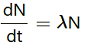Where,

Hence, the disintegration rate of 15 mg of the given isotope is
7.878 × 1010 atoms/s.

Question11. Obtain approximately the ratio of the nuclear radii of the gold isotope and the silver isotope.

Solution :
Nuclear radius of the gold isotope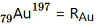Nuclear radius of the silver isotope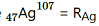Mass number of gold, AAu = 197

Mass number of silver, AAg = 107

The ratio of the radii of the two nuclei is related with their mass numbers as:

Hence, the ratio of the nuclear radii of the gold and silver isotopes is about 1.23.

Question12. Find the Q-value and the kinetic energy of the emitted α-particle in the α-decay of (a) and (b).

Given m = 226.02540 u,m = 222.01750 u,

= 220.01137 u, = 216.00189 u.

Solution :
(a) Alpha particle decay of emits a helium nucleus. As a result, its mass number reduces to (226 − 4) 222 and its atomic number reduces to (88 − 2) 86. This is shown in the following nuclear reaction.

Q-value of

emitted α-particle = (Sum of initial mass − Sum of final mass) c2

Where,

c = Speed of light

It is given that:

Q-value = [226.02540 − (222.01750 + 4.002603)] u c2
= 0.005297 u c2

But 1 u = 931.5 MeV/c2

∴Q = 0.005297 × 931.5 ≈ 4.94 MeV

Kinetic energy of the α-particle =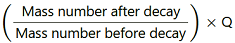= (222/226) x 4.94 = 4.85 MeV

(b) Alpha particle decay of is shown by the following nuclear reaction.

It is given that:

Mass of = 220.01137 u

Mass of = 216.00189 u

∴ Q-value = [220.01137 – (216.00189 +.00260)] × 931.5

≈ 641 MeV

Kinetic energy of the α-particle  = (220-4/220) x 6.41

= 6.29 MeV

Question13. The radionuclide 11C decays according to

The maximum energy of the emitted positron is 0.960 MeV.

Given the mass values:

calculate Q and compare it with the maximum energy of the positron emitted

Solution :
The given nuclear reaction is:

Atomic mass of m (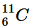) = 11.011434 u

Atomic mass of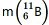= 11.009305 u

Maximum energy possessed by the emitted positron = 0.960 MeV

The change in the Q-value (ΔQ) of the nuclear masses of thenucleus is given as: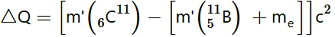….(i)

Where,

me = Mass of an electron or positron = 0.000548 u

c = Speed of light

m’ = Respective nuclear masses

If atomic masses are used instead of nuclear masses, then we have to add 6 me in the case of 11Cand 5 min the case of 11B.

Hence, equation (1) reduces to:

∴ΔQ = [11.011434 − 11.009305 − 2 × 0.000548] c2

= (0.001033 c2) u

But 1 u = 931.5 Mev/c2

∴ΔQ = 0.001033 × 931.5 ≈ 0.962 MeV

The value of Q is almost comparable to the maximum energy of the emitted positron.

Question14. The nucleus decays byemission. Write down the decay equation and determine the maximum kinetic energy of the electrons emitted. Given that:

= 22.994466 u

= 22.989770 u.

Solution :

Thedecay equation is

(we did not subtract the mass of the electron as it is cancelled because of the presence of one more electron in the sodium atom)

Q=0.004696931.5

Q=4.3743 eV

The emitted nucleus is way heavier than theparticle and the energy of the antineutrino is also negligible and therefore the maximum energy of the emitted electron is equal to the Q value.

Question15. The Q value of a nuclear reaction A + b → C + d is defined by

Q = [ mA+ mb− mC− md]cwhere the masses refer to the respective nuclei. Determine from the given data the Q-value of the following reactions and state whether the reactions are exothermic or endothermic.

(i)

(ii)

Atomic masses are given to be

Solution :
(i) The given nuclear reaction is:

It is given that:

According to the question, the Q-value of the reaction can be written as:

The negativeQ-value of the reaction shows that the reaction is endothermic.

(ii) The given nuclear reaction is:

It is given that:

The Q-value of this reaction is given as

The positive Q-value of the reaction shows that the reaction is exothermic.

Question 16:

Solution :
The fission of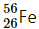can be given as:

It is given that:

The Q-value of this nuclear reaction is given as:

The Q-value of the fission is negative. Therefore, the fission is not possible energetically. For an energetically-possible fission reaction, the Q-value must be positive.

Solution :
Average energy released per fission of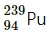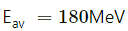Amount of pure, m = 1 kg = 1000 g

NA= Avogadro number = 6.023 × 1023

Mass number of= 239 g

Solution :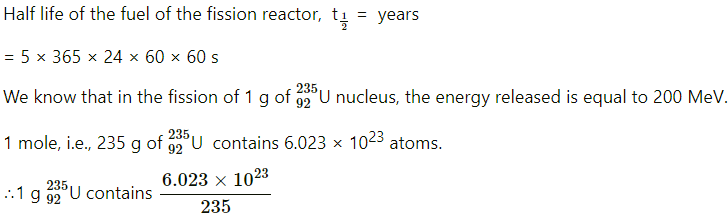Question19. How long can an electric lamp of 100W be kept glowing by fusion of 2.0 kg of deuterium? Take the fusion reaction as

Solution :
The given fusion reaction is:

Amount of deuterium, m = 2 kg

1 mole, i.e., 2 g of deuterium contains 6.023 × 1023 atoms.

It can be inferred from the given reaction that when two atoms of deuterium fuse, 3.27 MeV energy is released.

∴Total energy per nucleus released in the fusion reaction:

Question20. Calculate the height of the potential barrier for a head on collision of two deuterons. (Hint: The height of the potential barrier is given by the Coulomb repulsion between the two deuterons when they just touch each other. Assume that they can be taken as hard spheres of radius 2.0 fm.)

Solution :
When two deuterons collide head-on, the distance between their centres, d is given as:

Radius of a deuteron nucleus = 2 fm = 2 × 10−15 m

∴d = 2 × 10−15 + 2 × 10−15 = 4 × 10−15 m

Charge on a deuteron nucleus = Charge on an electron = e = 1.6 × 10−19 C

Potential energy of the two-deuteron system:

Question21. From the relation R = R0A1/3, where R0 is a constant and A is the mass number of a nucleus, show that the nuclear matter density is nearly constant (i.e. independent of A).

Solution :
We have the expression for nuclear radius as:

R = R0A1/3

Where,

R0 = Constant.

A = Mass number of the nucleus

Solution :

Question23

Solution :
Average atomic mass of magnesium, m = 24.312 u

Solution :

Question25. A source contains two phosphorous radio nuclides (T1/2 = 14.3d) and (T1/2 = 25.3d). Initially, 10% of the decays come from. How long one must wait until 90% do so?

Solution :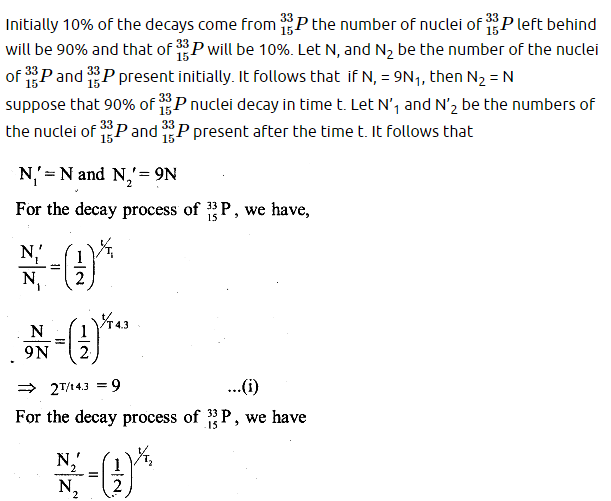Question26. Under certain circumstances, a nucleus can decay by emitting a particle more massive than an α-particle. Consider the following decay processes:

Calculate the Q-values for these decays and determine that both are energetically allowed.

Solution :
Take a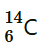emission nuclear reaction:

We know that:

Mass of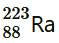m1 = 223.01850 u

Mass of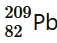m2 = 208.98107 u

Mass of, m3 = 14.00324 u

Hence, the Q-value of the reaction is given as:

Q = (m1 − m2 − m3) c2

= (223.01850 − 208.98107 − 14.00324) c2

= (0.03419 c2) u

But 1 u = 931.5 MeV/c2

∴Q = 0.03419 × 931.5

= 31.848 MeV

Hence, the Q-value of the nuclear reaction is 31.848 MeV. Since the value is positive, the reaction is energetically allowed.

Now take a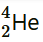emission nuclear reaction:

We know that:

Mass ofm1 = 223.01850

Mass of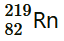m2 = 219.00948

Mass of, m3 = 4.00260

Q-value of this nuclear reaction is given as:

Q = (m1 − m2 − m3) c2

= (223.01850 − 219.00948 − 4.00260) C2

= (0.00642 c2) u

= 0.00642 × 931.5 = 5.98 MeV

Hence, the Q value of the second nuclear reaction is 5.98 MeV. Since the value is positive, the reaction is energetically allowed.

Question27. Consider the fission of by fast neutrons. In one fission event, no neutrons are emitted and the final end products, after the beta decay of the primary fragments, are and. Calculate Q for this fission process. The relevant atomic and particle masses are

m =238.05079 u

m =139.90543 u

m = 98.90594 u

Solution :
In the fission of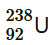, 10 β− particles decay from the parent nucleus. The nuclear reaction can be written as:

It is given that:

Mass of a nucleus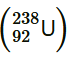m1 = 238.05079 u

Mass of a nucleus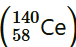m2 = 139.90543 u

Mass of a nucleus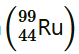, m3 = 98.90594 u

Mass of a neutron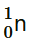m4 = 1.008665 u

Q-value of the above equation,

Where,

m’ = Represents the corresponding atomic masses of the nuclei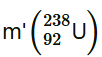= m1 − 92me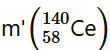= m2 − 58me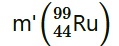= m3 − 44me

= m4

Hence, the Q-value of the fission process is 231.007 MeV.

Question28. Consider the D−T reaction (deuterium − tritium fusion)

(a) Calculate the energy released in MeV in this reaction from the data:

= 2.014102 u

= 3.016049 u

(b)Consider the radius of both deuterium and tritium to be approximately 2.0 fm. What is the kinetic energy needed to overcome the coulomb repulsion between the two nuclei? To what temperature must the gas be heated to initiate the reaction? (Hint: Kinetic energy required for one fusion event =average thermal kinetic energy available with the interacting particles = 2(3kT/2); k = Boltzman’s constant, T = absolute temperature.)

Solution :
(a) Take the D-T nuclear reaction: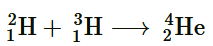It is given that:

Mass of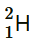, m1= 2.014102 u

Mass of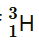, m= 3.016049 u

Mass of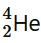m= 4.002603 u

Mass of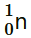, m= 1.008665 u

Q-value of the given D-T reaction is:

Q = [m+ m2− m3 − m4] c2

= [2.014102 + 3.016049 − 4.002603 − 1.008665] c2

= [0.018883 c2] u

But 1 u = 931.5 MeV/c2

∴Q = 0.018883 × 931.5 = 17.59 MeV

(b) Radius of deuterium and tritium, r ≈ 2.0 fm = 2 × 10−15 m

Distance between the two nuclei at the moment when they touch each other, d = r + r = 4 × 10−15 m

Charge on the deuterium nucleus = e

Charge on the tritium nucleus = e

Hence, the repulsive potential energy between the two nuclei is given as:

Where,

0 = Permittivity of free space

Hence, 5.76 × 10−14 J or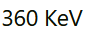of kinetic energy (KE) is needed to overcome the Coulomb repulsion between the two nuclei.

However, it is given that:

KE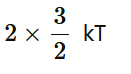Where,

k = Boltzmann constant = 1.38 × 10−23 m2 kg s−2 K−1

T = Temperature required for triggering the reaction

Hence, the gas must be heated to a temperature of 1.39 × 109 K to initiate the reaction.

Question29. Obtain the maximum kinetic energy of β-particles, and the radiation frequencies of γ decays in the decay scheme shown in Fig. 13.6. You are given that

m (198Au) = 197.968233 u

m (198Hg) =197.966760 u

Solution :
It can be observed from the given γ-decay diagram that γ1 decays from the 1.088 MeV energy level to the 0 MeV energy level.

Hence, the energy corresponding to γ1-decay is given as:

E1 = 1.088 − 0 = 1.088 MeV

1= 1.088 × 1.6 × 10−19 × 106 J

Where,

h = Planck’s constant = 6.6 × 10−34 Js

It can be observed from the given γ-decay diagram that γ2 decays from the 0.412 MeV energy level to the 0 MeV energy level.

Hence, the energy corresponding to γ2-decay is given as:

E2 = 0.412 − 0 = 0.412 MeV

2= 0.412 × 1.6 × 10−19 × 106 J

Where,

It can be observed from the given γ-decay diagram that γ3 decays from the 1.088 MeV energy level to the 0.412 MeV energy level.

Hence, the energy corresponding to γ3-decay is given as:

E3 = 1.088 − 0.412 = 0.676 MeV

3= 0.676 × 10−19 × 106 J

Where,

Mass of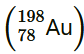= 197.968233 u

Mass of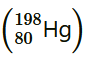= 197.966760 u

1 u = 931.5 MeV/c2

Energy of the highest level is given as:

βdecays from the 1.3720995 MeV level to the 1.088 MeV level

∴Maximum kinetic energy of the βparticle = 1.3720995 − 1.088

= 0.2840995 MeV

βdecays from the 1.3720995 MeV level to the 0.412 MeV level

∴Maximum kinetic energy of the βparticle = 1.3720995 − 0.412

= 0.9600995 MeV

Question30. Calculate and compare the energy released by a) fusion of 1.0 kg of hydrogen deep within Sun and b) the fission of 1.0 kg of 235U in a fission reactor.

Solution :
(a) Amount of hydrogen, m = 1 kg = 1000 g

1 mole, i.e., 1 g of hydrogen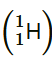contains 6.023 × 1023 atoms.

∴1000 g ofcontains 6.023 × 1023 × 1000 atoms.

Within the sun, fournuclei combine and form one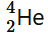nucleus. In this process 26 MeV of energy is released.

Hence, the energy released from the fusion of 1 kgis:

(b) Amount of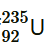= 1 kg = 1000 g

1 mole, i.e., 235 g ofcontains 6.023 × 1023 atoms.

∴1000 g ofcontains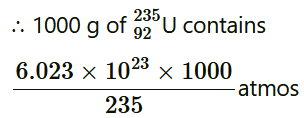It is known that the amount of energy released in the fission of one atom ofis 200 MeV.

Hence, energy released from the fission of 1 kg ofis:

Therefore, the energy released in the fusion of 1 kg of hydrogen is nearly 8 times the energy released in the fission of 1 kg of uranium.

Question31. Suppose India had a target of producing by 2020 AD, 200,000 MW of electric power, ten percent of which was to be obtained from nuclear power plants. Suppose we are given that, on an average, the efficiency of utilization (i.e. conversion to electric energy) of thermal energy produced in a reactor was 25%. How much amount of fissionable uranium would our country need per year by 2020? Take the heat energy per fission of 235U to be about 200MeV.

Solution :
Amount of electric power to be generated, P = 2 × 105 MW

10% of this amount has to be obtained from nuclear power plants.

∴Amount of nuclear power,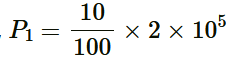= 2 × 104 MW

= 2 × 104 × 106 J/s

= 2 × 1010 × 60 × 60 × 24 × 365 J/y

Heat energy released per fission of a 235U nucleus, E = 200 MeV

Efficiency of a reactor = 25%

Hence, the amount of energy converted into the electrical energy per fission is calculated as:

Number of atoms required for fission per year:

1 mole, i.e., 235 g of U235 contains 6.023 × 1023 atoms.

∴Mass of 6.023 × 1023 atoms of U235 = 235 g = 235 × 10−3 kg

∴Mass of 78840 × 1024 atoms of U235

Hence, the mass of uranium needed per year is 3.076 × 104 kg.

Get 30% off your first purchase!

X
error: Content is protected !!
Scroll to Top